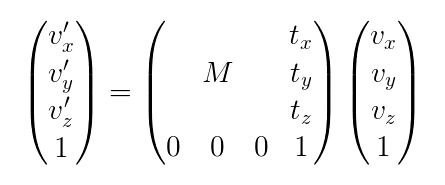## 12.4. Affine Transformations

For mapping e.g., world to voxel coordinates, or device to world coordinates, affine transformations have to be applied. This is done with homogeneous coordinates:

• Extend the (x,y,z) triple by an artificial coordinate with a fixed value 1.

• Affine transforms can then be represented by a single matrix multiplication.

Why not a 3x3 matrix? Two reasons:

1. One cannot construct a 3x3 matrix that will translate the point (0,0,0). The zeroes in the coordinate vector cancel out all the coefficients.

2. Transformations could not be combined by multiplying the matrices.

Affine transformations have these elementary transforms:

• Translation (moves an object along a direction vector)

• Rotation (rotates the object around an axis vector)

• Scaling (shrinks/grows the object size)

• Shearing (deforms the object; rare in medical image data)

Figure 12.3. Matrix MultiplicationTipLook at the example Chapter 5, Defining a Region of Interest (ROI) for the module `WorldToVoxel` in action.

The voxel coordinate system is a continuous coordinate system. Voxel boundaries are at integer values, voxel centers are 0.5 off. To transform integer voxel indices to voxel centers in world coordinates, either add the value “0.5” to voxel indices or check the option Integer Voxel Coordinates in the modules `WorldVoxelConvert`, `SoMLTransform`, and others.

Common pitfalls

• Computing the voxel volume: getVoxelSize() returns voxel spacing in x, y and z. The product of these values is not the voxel volume if the voxel-to-world-matrix is not orthogonal. Solution: Use the absolute value of the matrix determinant instead.

• Inventor using row vector conventions: ML and MeVisLab use the widespread column vector conventions, that is vectors are written as columns and matrices are applied by left-multiplication. Open Inventor, in contrast, uses row vector conventions, that is vectors are written as rows and matrices are applied by right-multiplication. Solution: Use the matrix transposition to convert a matrix from one convention to the other.